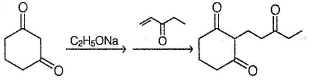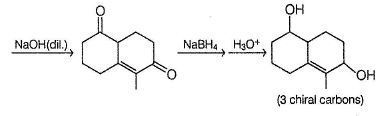Courses

# Test: Unsaturated Aldehydes & Ketones

## 15 Questions MCQ Test Chemistry Class 12 | Test: Unsaturated Aldehydes & Ketones

Description
This mock test of Test: Unsaturated Aldehydes & Ketones for Class 12 helps you for every Class 12 entrance exam. This contains 15 Multiple Choice Questions for Class 12 Test: Unsaturated Aldehydes & Ketones (mcq) to study with solutions a complete question bank. The solved questions answers in this Test: Unsaturated Aldehydes & Ketones quiz give you a good mix of easy questions and tough questions. Class 12 students definitely take this Test: Unsaturated Aldehydes & Ketones exercise for a better result in the exam. You can find other Test: Unsaturated Aldehydes & Ketones extra questions, long questions & short questions for Class 12 on EduRev as well by searching above.
QUESTION: 1

### Only One Option Correct Type Direction (Q. Nos. 1-5) This section contains 5 multiple choice questions. Each question has four choices (a), (b), (c) and (d), out of which ONLY ONE is correct. Q.  Predict the major product in the given reaction,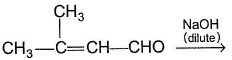Solution: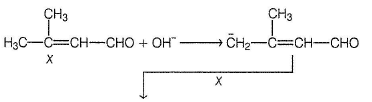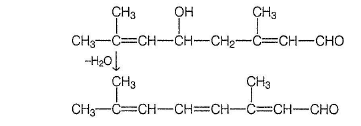QUESTION: 2

### The major organic product in the following reaction is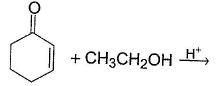Solution: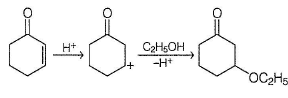QUESTION: 3

### What is the major product in the following reaction ?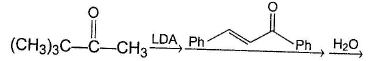Solution: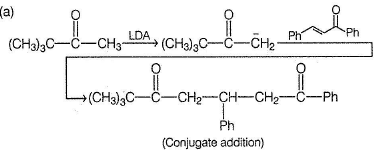QUESTION: 4

The major organic product in the following reaction is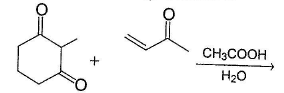Solution: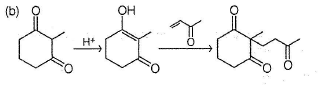QUESTION: 5

In the reaction given below,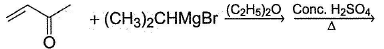Q.

The final major product is

Solution: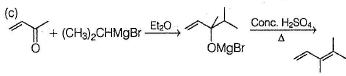*Multiple options can be correct
QUESTION: 6

One or More than One Options Correct Type

Direction (Q. Nos. 6-9) This section contains 4 multiple choice questions. Each question has four choices (a), (b), (c) and (d), out of which ONE or MORE THAN ONE are correct.

In the reaction given below,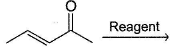Q.

which reagent(s) below would undergo addition at olefinic bond?

Solution:

Weak nucleophiles (Cl , NH3) undergo conjugate addition. Strong nucleophiles (R-, H-) undergo direct addition.

*Multiple options can be correct
QUESTION: 7

In the reaction given below,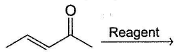Q.

Solution:

Strong nucleophiles (CH3MgBr, CH3Li) undergo direct addition.

*Multiple options can be correct
QUESTION: 8

What is/are true about nucleophilic addition reaction at α, β-unsaturated carbonyl compound of type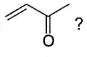Solution:

Strong nucleophiles (like H- from LiAIH4) un dergo direct addition while weak nucleophiles (like R2CuLi, RNH2etc.) undergo conjugate addition at olefinic bond.

*Multiple options can be correct
QUESTION: 9

Consider the following reaction,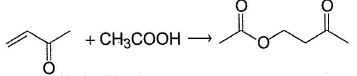Q.

The correct stalement(s) regarding mechanism of the above reaction and the reaction outcome is/are

Solution:

CH3COOH undergoes nucleophilic addition at conjugate position giving indicated product as major one.

QUESTION: 10

Comprehension Type

Direction (Q. Nos. 10-12) This section contains a paragraph, describing theory, experiments, data, etc.
Three questions related to the paragraph have been given. Each question has only one correct answer among the four given options (a), (b), (c) and (d).

Passage

Consider the following multistep synthesis :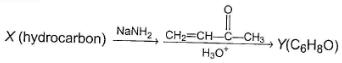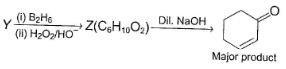Q.

The hydrocarbon X is

Solution:

Y has 2 carbon extra than unsaturated ketone, hence starting compound must be acetylene and nucleophile undergoing conjugate addition is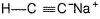QUESTION: 11

Consider the following multistep synthesis :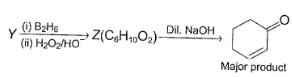Q.

The structure of compound Y is

Solution: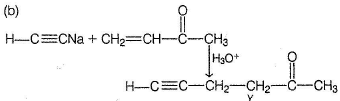QUESTION: 12

Consider the following multistep synthesis :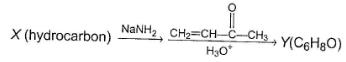Q.

If X is replaced by pentane -2 ,4 -dione, product equivalent to Y would be

Solution: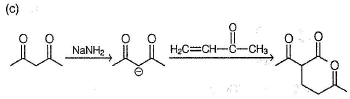*Answer can only contain numeric values
QUESTION: 13

One Integer Value Correct Type

Direction (Q. Nos. 13-15) This section contains 3 questions. When worked out will result in an integer from 0 to 9 (both inclusive).

Q.

In the reaction given below, how many different stereoisomers of major product are possible?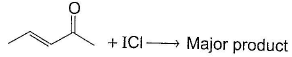Solution: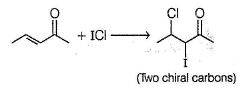*Answer can only contain numeric values
QUESTION: 14

In the reaction given as,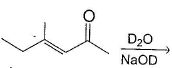some of the hydrogen undergo exchange by deuterium. At the most, how many deuterium atoj*is incorporation are possible per molecule of keton ?

Solution: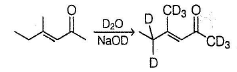*Answer can only contain numeric values
QUESTION: 15

Consider the following sequence of reaction,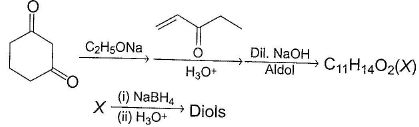Q.

How many different stereoisomers of diol are formed ?

Solution: